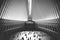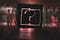# Mathematics behind Gradient Descent..Simply ExplainedPhoto by Adam Bentley on Unsplash

So far we have discussed linear regression and gradient descent in previous articles. We got a simple overview of the concepts and a practical tutorial to understand how they work. In this article, we will see the mathematics behind gradient descent and how can an “optimizer” get the global minima point. If the term “optimizer” is new for you, it is simply the function that works to determine the global minima point which refers to the coefficients of best-fit line in linear regression algorithm. By the way, similar concepts are used in deep learning algorithms. …

# Gradient Descent..Simply Explained With A Tutorial

In the previous blog Linear Regression, A general overview was given about simple linear regression. Now it’s time to know how to train your simple linear regression model and how to get the line that fits your data set.

Gradient Descent is simply a technique to find the point of minimum error (Sum of squared residuals), which represents the coefficient (a) and intercept (b) of the best-fit line in the line equation y=ax+b

Let’s re-invent the wheel to determine the coefficients of our linear regression model with few lines of code. …

# Linear Regression

Understanding linear regression concepts well is the best way to establish a solid base for all machine learning and deep learning algorithms, so even you have long experience in deploying advanced ML/DL models, it is good to refresh your basics and maintain your structures. The goal of linear regression models is to predict or forecast an independent random variable based on dependent variables. For example, if we have a data set of salaries of a group of engineers based on their years of experience. Our task is to train a model with our data set so that it can predict…

# How to Understand A Confusion Matrix?Photo by Emily Morter on Unsplash

After fitting your data to a ML algorithm, you have a predictions of the independent variable. Now you need to evaluate how well you model is. In classification problems, we need to build a model that is able to classify the data in a way that suits our use case. In this blog we will view the confusion matrix. How to be understood and interpreted.

First, assume that the classification problem we have is binary which means that the prediction of our model is 1 or 0. If the predicted output is belong to that class or not. …